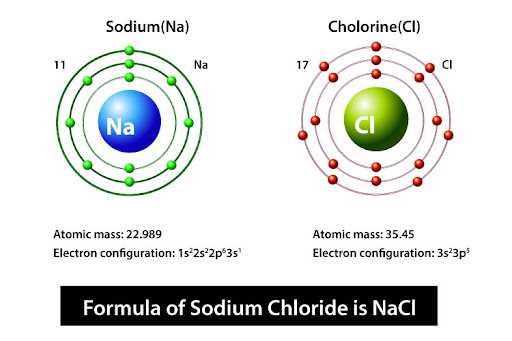# The valency of sodium is 1. The valency of chlorine is 1. Write the formula of sodium chloride.

The formula of Sodium Chloride is NaCl
Explanation:
Sodium (Na) has a valency of 1 because it has only one valence electron in the outermost shell (3s1). There are weak attractive forces between this electron and the nucleus so it acts as a free electron. It can easily be removed by applying energy called Ionizationation energy. Once this electron is removed Na becomes Na+ ion and is more stable because it has a complete octet in its outermost shell i.e. (2s2 2p6)
Chlorine on the other hand has 7 valence electrons and it requires one more to achieve a stable configuration. The electron from sodium is gained by chlorine so Cl becomes Cl- ion. The electrostatic forces of attraction between Na+ and Cl- result in an ionic bond. So chlorine also has a valency of 1.
Valency is the bonding power of an element and chemical formula is devised by swapping the valency of each element on their Chemical Symbols.
In the case of Sodium Chloride, the Valency is 1 each so we don't need to mention it in the formula. I.e. NaCl
Note:
Compounds in general do not possess valencies, it is the ions or the combining atoms making up the compound that have different valencies and these valencies give rise to different chemical formulae for different components. For ions, the valency is equal to the charge possessed by the molecule. For covalent bonds, the Valency is equal to the combing capacity of each atom.
e.g in water, oxygen has a Valency of 2 and hydrogen has 1. So the chemical formula for water is H20. Thus, oxygen can combine with 2 molecules of hydrogen because it has the combining capacity i.e Valency of 2.